## RD Sharma Solutions for Class 6 Chapter 5 Negative Numbers and Integers Free Online

Exercise 5.1 PAGE: 5.5
1. Write the opposite of each of the following:
(i) Increase in population
(ii) Depositing money in a bank
(iii) Earning money
(iv) Going North
(v) Gaining a weight of 4kg
(vi) A loss of Rs 1000
(vii) 25
(viii) – 15
Solution:
(i) The opposite of Increase in population is Decrease in population.
(ii) The opposite of Depositing money in a bank is Withdrawing money from a bank.
(iii) The opposite of earning money is Spending money.
(iv) The opposite of Going North is Going South.
(v) The opposite of gaining a weight of 4kg is losing a weight of 4kg.
(vi) The opposite of a loss of Rs 1000 is a gain of Rs 1000.
(vii) The opposite of 25 is – 25.
(viii) The opposite of – 15 is 15.
2. Indicate the following by using integers:
(i) 25o above zero
(ii) 5o below zero
(iii) A profit of Rs 800
(iv) A deposit of Rs 2500
(v) 3km above sea level
(vi) 2km below level
Solution:
(i) 25o above zero is + 25o.
(ii) 5o below zero is – 5o.
(iii) A profit of Rs 800 is + 800.
(iv) A deposit of Rs 2500 is + 2500.
(v) 3km above sea level is + 3.
(vi) 2km below level is – 2.
3. Mark the following integers on a number line:
(i) 7
(ii) -4
(iii) 0
Solution:
The following integers are marked on a number line as given below: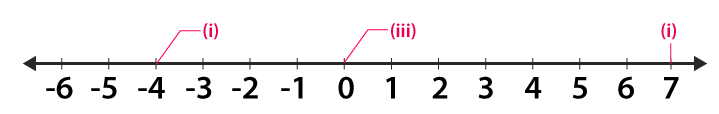4. Which number in each of the following pairs is smaller?
(i) 0, -4
(ii) -3 , 12
(iii) 8, 13
(iv) – 15, -27
Solution:
(i) 0 is greater than the negative integers
So we get – 4 < 0
Therefore, – 4 is smaller.
(ii) 12 is greater than -3 on a number line
So we get
-3 < 12
Therefore, – 3 is smaller.
(iii) 13 is greater than 8 on a number line
So we get 8 < 13
Therefore, 8 is smaller.
(iv) – 15 is greater than – 27 on a number line
So we get – 27 < – 15
Therefore, – 27 is smaller.
5. Which number in each of the following pairs is larger?
(i) 3, -4
(ii) – 12, – 8
(iii) 0, 7
(iv) 12, – 18
Solution:
(i) We know that 3 is larger than – 4 on a number line
So we get 3 > – 4
Therefore, 3 is larger.
(ii) We know that – 8 is larger than – 12 on a number line
So we get – 8 > – 12
Therefore, – 8 is larger.
(iii) We know that 7 is larger than 0 on a number line
So we get 7 > 0
Therefore, 7 is larger.
(iv) We know that 12 is larger than – 18 on a number line
So we get 12 > – 18
Therefore, 12 is larger.
6. Write all integers between:
(i) – 7 and 3
(ii) – 2 and 2
(iii) – 4 and 0
(iv) 0 and 3
Solution:
(i) The integers between – 7 and 3 are
– 6, – 5, – 4, – 3, – 2, – 1, 0, 1, 2
(ii) The integers between – 2 and 2 are
-1, 0, 1.
(iii) The integers between – 4 and 0 are
-3, -2, -1
(iv) The integers between 0 and 3 are
1, 2.
7. How many integers are between?
(i) – 4 and 3
(ii) 5 and 12
(iii) – 9 and – 2
(iv) 0 and 5
Solution:
(i) The integers between – 4 and 3 are
-3, -2, -1, 0, 1, 2
Therefore, number of integers between – 4 and 3 are 6.
(ii) The integers between 5 and 12 are
6, 7, 8, 9, 10, 11
Therefore, number of integers between 5 and 12 are 6.
(iii) The integers between – 9 and – 2 are
-8, -7, -6, -5, -4, -3
Therefore, number of integers between -9 and -2 are 6.
(iv) The integers between 0 and 5 are
1, 2, 3, 4
Therefore, number of integers between 0 and 5 are 4.
8. Replace * in each of the following by < or > so that the statement is true:
(i) 2 * 5
(ii) 0 * 3
(iii) 0 * – 7
(iv) – 18 * 15
(v) – 235 * – 532
(vi) – 20 * 20
Solution:
(i) 2 < 5
(ii) 0 < 3
(iii) 0 > – 7
(iv) – 18 < 15
(v) – 235 > – 532
(vi) – 20 < 20
9. Write the following integers in increasing order:
(i) – 8, 5, 0, -12, 1, -9, 15
(ii) – 106, 107, – 320, – 7, 185
Solution:
(i) – 8, 5, 0, -12, 1, -9, 15 can be written in increasing order as
– 12, – 9, – 8, 0, 1, 5, 15
(ii) – 106, 107, – 320, – 7, 185 can be written in increasing order as
-320, – 106, – 7, 107, 185.
10. Write the following integers in decreasing order:
(i) – 15, 0, -2, -9, 7, 6, -5, 8
(ii) -154, 123, -205, -89, -74
Solution:
(i) – 15, 0, -2, -9, 7, 6, -5, 8 can be written in decreasing order as
8, 7, 6, 0, -2, -5, -9, -15
(ii) -154, 123, -205, -89, -74 can be written in decreasing order as
123, – 74, – 89, – 154, – 205
11. Using the number line, write the integer which is:
(i) 2 more than 3
(ii) 5 less than 3
(iii) 4 more than – 9
Solution:
(i) 2 more than 3
In order to get the integer 2 more than 3
We draw a number line from 3 and proceed 2 units to the right to obtain 5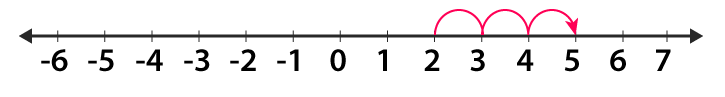Therefore, 2 more than 3 is 5.
(ii) 5 less than 3
In order to get the integer 5 less than 3
We draw a number line from 3 and proceed 5 units to the left to obtain – 2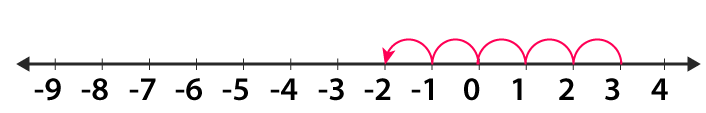Therefore, 5 less than 3 is – 2.
(iii) 4 more than – 9
In order to get the integer 4 more than – 9
We draw a number line from – 9 and proceed 4 units to the right to obtain -5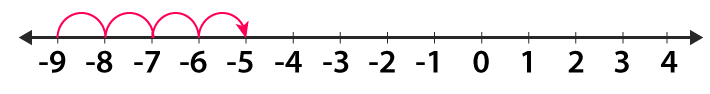Therefore, 4 more than – 9 is – 5.
12. Write the absolute value of each of the following:
(i) 14
(ii) – 25
(iii) 0
(iv) – 125
(v) – 248
(vi) a – 7, if a is greater than 7
(vii) a – 7, if a – 2 is less than 7
(viii) a + 4, if a is greater than -4
(ix) a + 4 if a is less than – 4
(x) |-3|
(xi) -|-5|
(xii) |12 – 5|
Solution:
(i) The absolute value of 14 is
|14| = 14
(ii) The absolute value of – 25 is
|-25| = 25
(iii) The absolute value of 0 is
|0| = 0
(iv) The absolute value of – 125 is
|-125| = 125
(v) The absolute value of – 248 is
|-248| = 248
(vi) The absolute value of a – 7, if a is greater than 7 is
|a – 7| = a – 7 where a > 7
(vii) The absolute value of a – 7, if a – 2 is less than 7 is
|a – 7| = – (a – 7) where a – 2 < 7
(viii) The absolute value of a + 4, if a is greater than -4 is
|a + 4| = a + 4 where a > – 4
(ix) The absolute value of a + 4 if a is less than – 4 is
|a + 4| = – (a + 4) where a < -4
(x) The absolute value of |-3| is
|-3| = 3
(xi) The absolute value of -|-5| is
-|-5| = 5
(xii) The absolute value of |12 – 5| is
|12 – 5| = 7
13. (i) Write 4 negative integers less than – 10.
(ii) Write 6 negative integers just greater than – 12.
Solution:
(i) The 4 negative integers less than – 10 are
– 11, – 12, – 13, – 14
(ii) The 6 negative integers just greater than – 12 are
-11, – 10, – 9, – 8, – 7, – 6
14. Which of the following statements are true?
(i) The smallest integer is zero.
(ii) The opposite of zero is zero.
(iii) Zero is not an integer.
(iv) 0 is larger than every negative integer.
(v) The absolute value of an integer is greater than the integer.
(vi) A positive integer is greater than its opposite.
(vii) Every negative integer is less than every natural number.
(viii) 0 is the smallest positive integer.
Solution:
(i) False. The smallest integer is 1.
(ii) True. 0 is neither positive nor negative so the opposite is 0.
(iii) False. Zero is an integer which is neither positive nor negative.
(iv) True. 0 is larger than – 1.
(v) False. The absolute value of an integer is the numerical value.
(vi) True. 3 is greater than – 3.
(vii) True. – 3 is less than 1.
(viii) False. 1 is the smallest positive integer.

Exercise 5.2 PAGE: 5.9
1. Draw a number line and represent each of the following on it:
(i) 5 + (-2)
(ii) (-9) + 4
(iii) (-3) + (-5)
(iv) 6 + (-6)
(v) (-1) + (-2) + 2
(vi) (-2) + 7 + (-9)
Solution:
(i) 5 + (-2)
From 0 move towards right of first five units to obtain + 5
So the second number is – 2 so move 2 units towards left of + 5 we get + 3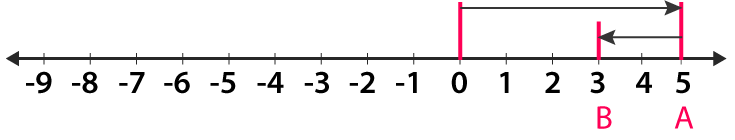Therefore, 5 + (-2) = 3.
(ii) (-9) + 4
From 0 move towards left of nine units to obtain – 9
So the second number is 4 so move 4 units towards right of – 9 we get – 5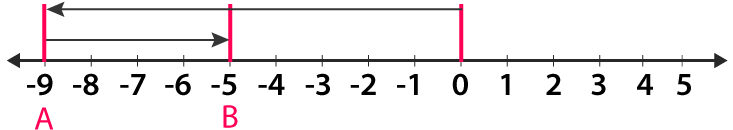Therefore, (-9) + 4 = – 5.
(iii) (-3) + (-5)
From 0 move towards left of three units to obtain – 3
So the second number is – 5 so move 5 units towards left of – 3 we get – 8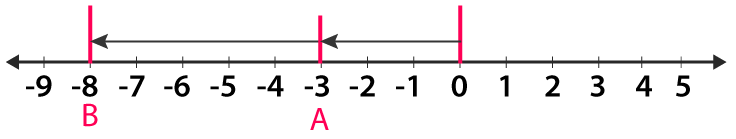Therefore, (-3) + (-5) = – 8.
(iv) 6 + (-6)
From zero move towards right of six units to obtain 6
So the second number is – 6 so move 6 units towards left of 6 we get 0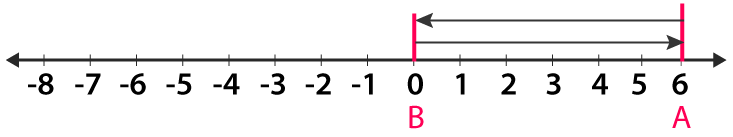Therefore, 6 + (-6) = 0.
(v) (-1) + (-2) + 2
From zero move towards left of one unit to obtain – 1
So the second number is – 2 so move 2 units towards left of – 1 we get – 3
The third number is 2 so move 2 units towards right of – 3 we get – 1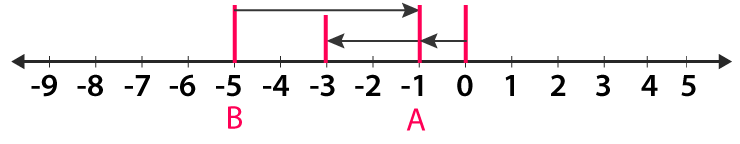Therefore, (-1) + (-2) + 2 = – 1.
(vi) (-2) + 7 + (-9)
From zero move towards left of two units to obtain – 2
So the second number is 7 so move 7 units towards right of – 2 we get 5
The third number is – 9 so move 9 units towards left of 5 we get – 4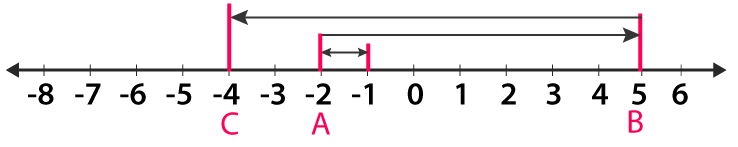Therefore, (-2) + 7 + (-9) = – 4.
2. Find the sum of
(i) -557 and 488
(ii) -522 and -160
(iii) 2567 and – 325
(iv) -10025 and 139
(v) 2547 and -2548
(vi) 2884 and -2884
Solution:
(i) -557 and 488
We get
-557 + 488
It can be written as
|-557| – |488| = 557 – 488 = – 69.
(ii) -522 and -160
We get
-522 + (-160)
It can be written as
-522 – 160 = – 682
(iii) 2567 and – 325
We get
2567 + (-325)
It can be written as
2567 – 325 = 2242
(iv) -10025 and 139
We get
-10025 + 139
It can be written as
-10025 + 139 = -9886
(v) 2547 and -2548
We get
2547 + (-2548)
It can be written as
2547 – 2548 = -1
(vi) 2884 and -2884
We get
2884 + (-2884)
It can be written as
2884 – 2884 = 0

Exercise 5.3 page: 5.11
1. Find the additive inverse of each of the following integers:
(i) 52
(ii) – 176
(iii) 0
(iv) 1
Solution:
(i) The additive inverse of 52 is – 52.
(ii) The additive inverse of – 176 is 176.
(iii) The additive inverse of 0 is 0.
(iv) The additive inverse of 1 is – 1.
2. Find the successor of each of the following integers:
(i) – 42
(ii) -1
(iii) 0
(iv) – 200
(v) -99
Solution:
(i) The successor of – 42 = – 42 + (-1)
We get
= 1 – 42 = – 41
(ii) The successor of – 1 is
-1 + 1 = 0
(iii) The successor of 0 is
0 + 1 = 1
(iv) The successor of – 200 is
-200 + 1 = – 199
(v) The successor of – 99 is
– 99 + 1 = – 98
3. Find the predecessor of each of the following integers:
(i) 0
(ii) 1
(iii) – 1
(iv) – 125
(v) 1000
Solution:
(i) The predecessor of 0 is
0 – 1 = – 1
(ii) The predecessor of 1 is
1 – 1 = 0
(iii) The predecessor of -1 is
-1 – 1 = -2
(iv) The predecessor of – 125 is
-125 – 1 = – 126
(v) The predecessor of 1000 is
1000 – 1 = 999
4. Which of the following statements are true?
(i) The sum of a number and its opposite is zero.
(ii) The sum of two negative integers is a positive integer.
(iii) The sum of a negative integer and a positive integer is always a negative integer.
(iv) The successor of – 1 is 1.
(v) The sum of three different integers can never be zero.
Solution:
(i) True. 1 – 1 = 0
(ii) False. -1 – 1 = -2
(iii) False. – 2 + 3 = 1
(iv) False. The successor of – 1 is 0.
(v) False. 1 + 2 – 3 = 0
5. Write all integers whose absolute values are less than 5.
Solution:
The integers whose absolute values are less than 5 are
-4, – 3, – 2, – 1, 0, 1, 2, 3, 4
6. Which of the following is false:
(i) |4 + 2| = |4| + |2|
(ii) |2 – 4| = |2| + |4|
(iii) |4 – 2| = |4| – |2|
(iv) |(-2) + (-4)| = |-2| + |-4|
Solution:
(i) True.
(ii) False.
(iii) True.
(iv) True.
7. Complete the following table: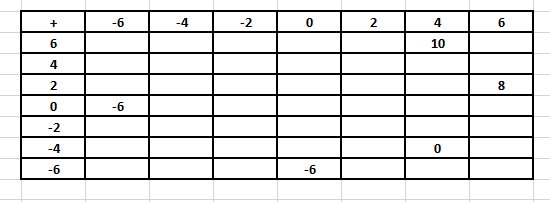From the above table:
(i) Write all the pairs of integers whose sum is 0.
(ii) Is (-4) + (-2) = (-2) + (-4)?
(iii) Is 0 + (-6) = -6?
Solution: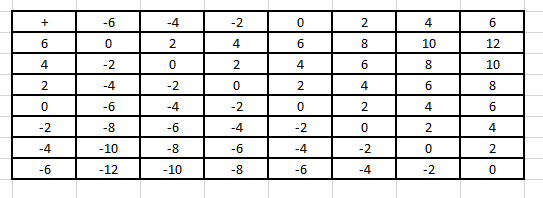(i) The pairs of integers whose sum is 0 are
(6, -6), (4, – 4), (3, – 3), (2, – 2), (1, – 1), (0, 0)
(ii) Yes. By using commutativity of addition (-4) + (-2) = (-2) + (-4)
(iii) Yes. By using additive identity 0 + (-6) = -6.
8. Find an integer x such that
(i) x + 1 = 0
(ii) x + 5 = 0
(iii) – 3 + x = 0
(iv) x + (-8) = 0
(v) 7 + x = 0
(vi) x + 0 = 0
Solution:
(i) x + 1 = 0
Subtracting 1 on both sides
x + 1 – 1 = 0 – 1
We get
x = -1
(ii) x + 5 = 0
By subtracting 5 on both sides
x + 5 – 5 = 0 – 5
So we get
x = -5
(iii) – 3 + x = 0
By adding 3 on both sides
-3 + x + 3 = 0 + 3
So we get
x = 3
(iv) x + (-8) = 0
By adding 8 on both sides
x – 8 + 8 = 0 + 8
So we get
x = 8
(v) 7 + x = 0
By subtracting 7 on both sides
7 + x – 7 = 0 – 7
So we get
x = – 7
(vi) x + 0 = 0
So we get
x = 0

Exercise 5.4 page: 5.17
1. Subtract the first integer from the second in each of the following:
(i) 12, -5
(ii) – 12, 8
(iii) – 225, – 135
(iv) 1001, 101
(v) – 812, 3126
(vi) 7560, – 8
(vii) – 3978, – 4109
(viii) 0, – 1005
Solution:
(i) 12, -5
So by subtracting the first integer from the second
-5 – 12 = – 17
(ii) – 12, 8
So by subtracting the first integer from the second
8 – (-12) = 8 + 12 = 20
(iii) – 225, – 135
So by subtracting the first integer from the second
-135 – (-225) = 225 – 135 = 90
(iv) 1001, 101
So by subtracting the first integer from the second
101 – 1001 = – 900
(v) – 812, 3126
So by subtracting the first integer from the second
3126 – (-812) = 3126 + 812 = 3938
(vi) 7560, – 8
So by subtracting the first integer from the second
-8 – 7560 = – 7568
(vii) – 3978, – 4109
So by subtracting the first integer from the second
-4109 – (-3978) = – 4109 + 3978 = -131
(viii) 0, – 1005
So by subtracting the first integer from the second
-1005 – 0 = – 1005
2. Find the value of:
(i) – 27 – (- 23)
(ii) – 17 – 18 – (-35)
(iii) – 12 – (-5) – (-125) + 270
(iv) 373 + (-245) + (-373) + 145 + 3000
(v) 1 + (-475) + (-475) + (-475) + (-475) + 1900
(vi) (-1) + (-304) + 304 + 304 + (-304) + 1
Solution:
(i) – 27 – (- 23)
So we get
= – 27 + 23
On further calculation
= 23 – 27
We get
= – 4
(ii) – 17 – 18 – (-35)
So we get
= – 35 + 35
On further calculation
= 0
(iii) – 12 – (-5) – (-125) + 270
So we get
= – 12 + 5 + 125 + 270
On further calculation
= 400 – 12
We get
= 388
(iv) 373 + (-245) + (-373) + 145 + 3000
So we get
= 373 – 245 – 373 + 145 + 3000
On further calculation
= 3145 + 373 – 373 – 245
We get
= 3145 – 245
By subtraction
= 2900
(v) 1 + (-475) + (-475) + (-475) + (-475) + 1900
So we get
= 1 – 950 – 950 + 1900
On further calculation
= 1900 + 1 – 1900
We get
= 1
(vi) (-1) + (-304) + 304 + 304 + (-304) + 1
So we get
= – 1 + 1 – 304 + 304 – 304 + 304
On further calculation
= 0
3. Subtract the sum of – 5020 and 2320 from – 709.
Solution:
We know that the sum of 5020 and 2320 is
-5020 + 2320
It can be written as
= 2320 – 5020
So we get
= – 2700
Subtracting – 709 we get
= – (-2700) + (-709)
On further calculation
= – 709 – (-2700)
We get
= – 709 + 2700
By subtraction
= 1991
4. Subtract the sum of – 1250 and 1138 from the sum of 1136 and – 1272.
Solution:
We know that the sum of – 1250 and 1138 is
-1250 + 1138
It can be written as
= 1138 – 1250
So we get
= – 112
We know that the sum of 1136 and – 1272 is
1136 – 1272 = – 136
So we get
-136 – (-112) = – 136 + 112 = -24
5. From the sum of 233 and – 147, subtract – 284.
Solution:
We know that the sum of 233 and – 147 is
233 – 147 = 86
Subtracting – 284 we get
86 – (-284) = 86 + 284 = 370
6. The sum of two integers is 238. If one of the integers is – 122, determine the other.
Solution:
It is given that
Sum of two integers = 238
One of the integers = – 122
So the other integer = – (-122) + 138
On further calculation
Other integer = 238 + 122 = 360
7. The sum of two integers is – 223. If one of the integers is 172, find the other.
Solution:
It is given that
Sum of two integers = – 223
One of the integers = 172
So the other integer = – 223 – 172 = – 395
8. Evaluate the following:
(i) – 8 – 24 + 31 – 26 – 28 + 7 + 19 – 18 – 8 + 33
(ii) – 26 – 20 + 33 – (-33) + 21 + 24 – (-25) – 26 – 14 – 34
Solution:
(i) – 8 – 24 + 31 – 26 – 28 + 7 + 19 – 18 – 8 + 33
We get
= – 8 – 24 – 26 – 28 – 18 – 8 + 31 + 7 + 19 + 33
On further calculation
= – 32 – 26 – 28 – 26 + 38 + 19 + 33
It can be written as
= 38 – 32 – 26 – 28 + 33 – 26 + 19
So we get
= 6 – 26 – 28 + 7 + 19
By calculation
= 6 – 28 – 26 + 26
= 6 – 28
By subtraction
= – 22
(ii) – 26 – 20 + 33 – (-33) + 21 + 24 – (-25) – 26 – 14 – 34
We get
= – 46 + 33 + 33 + 21 + 24 + 25 – 26 – 14 – 34
On further calculation
= – 46 + 66 + 21 + 24 + 25 + (-74)
It can be written as
= – 46 + 66 + 70 – 74
So we get
= – 46 – 4 + 66
By calculation
= – 50 + 66
= 66 – 50
By subtraction
= 16
9. Calculate
1 – 2 + 3 – 4 + 5 – 6 + ……… + 15 – 16
Solution:
It can be written as
1 – 2 + 3 – 4 + 5 – 6 + 7 – 8 + 9 – 10 + 11 – 12 + 13 – 14 + 15 – 16
We get
= – 1 – 1 – 1 – 1 – 1 – 1 – 1 – 1
By calculation
= – 8
10. Calculate the sum:
5 + (-5) + 5 + (-5) + …..
(i) if the number of terms is 10.
(ii) if the number of terms is 11.
Solution:
(i) if the number of terms is 10
We get
5 + (-5) + 5 + (-5) + 5 + (-5) + 5 + (-5) + 5 + (-5)
On further calculation
= 5 – 5 + 5 – 5 + 5 – 5 + 5 – 5 + 5 – 5 = 0
(ii) if the number of terms is 11
We get
5 + (-5) + 5 + (-5) + 5 + (-5) + 5 + (-5) + 5 + (-5) + 5
On further calculation
= 5 – 5 + 5 – 5 + 5 – 5 + 5 – 5 + 5 – 5 + 5 = 5
11. Replace * by < or > in each of the following to make the statement true:
(i) (-6) + (-9) * (-6) – (-9)
(ii) (-12) – (-12) * (-12) + (-12)
(iii) (-20) – (-20) * 20 – (65)
(iv) 28 – (-10) * (-16) – (-76)
Solution:
(i) (-6) + (-9) < (-6) – (-9)
(ii) (-12) – (-12) > (-12) + (-12)
(iii) (-20) – (-20) > 20 – (65)
(iv) 28 – (-10) < (-16) – (-76)
12. If △ is an operation on integers such that a △ b = – a + b – (-2) for all integers a, b. Find the value of
(i) 4 △ 3
(ii) (-2) △ (-3)
(iii) 6 △ (-5)
(iv) (-5) △ 6
Solution:
(i) 4 △ 3
By substituting values in a △ b = – a + b – (-2)
We get
4 △ 3 = – 4 + 3 – (-2) = 1
(ii) (-2) △ (-3)
By substituting values in a △ b = – a + b – (-2)
We get
(-2) △ (-3) = – (-2) + (-3) – (-2) = 1
(iii) 6 △ (-5)
By substituting values in a △ b = – a + b – (-2)
We get
6 △ (-5) = – 6 + (-5) – (-2) = – 9
(iv) (-5) △ 6
By substituting values in a △ b = – a + b – (-2)
We get
(-5) △ 6 = – (-5) + 6 – (-2) = 13
13. If a and b are two integers such that a is the predecessor of b. Find the value of a – b.
Solution:
It is given that a is the predecessor of b
We can write it as
a + 1 = b
So we get
a – b = – 1
14. If a and b are two integers such that a is the successor of b. Find the value of a – b.
Solution:
It is given that a is the successor of b
We can write it as
a – 1 = b
So we get
a – b = 1
15. Which of the following statements are true:
(i) – 13 > – 8 – (-2)
(ii) – 4 + (-2) < 2
(iii) The negative of a negative integer is positive.
(iv) If a and b are two integers such that a > b, then a – b is always a positive integer.
(v) The difference of two integers is an integer.
(vi) Additive inverse of a negative integer is negative.
(vii) Additive inverse of a positive integer is negative.
(viii) Additive inverse of a negative integer is positive.
Solution:
(i) False.
(ii) True.
(iii) True.
(iv) True.
(v) True.
(vi) False.
(vii) True.
(viii) True.
16. Fill in the blanks:
(i) – 7 + ….. = 0
(ii) 29 + ….. = 0
(iii) 132 + (-132) = ….
(iv) – 14 + ….. = 22
(v) – 1256 + ….. = – 742
(vi) ….. – 1234 = – 4539
Solution:
(i) – 7 + 7 = 0
(ii) 29 + (-29) = 0
(iii) 132 + (-132) = 0
(iv) – 14 + 36 = 22
(v) – 1256 + 514 = – 742
(vi) -3305 – 1234 = – 4539
Objective Type Questions page: 5.18
Mark the correct alternative in each of the following:
1. Which of the following statement is true?
(a) − 7 > − 5              (b) − 7 < − 5                 (c) (− 7) + (− 5) > 0                       (d) (− 7) − (− 5) > 0
Solution:
The option (b) is correct answer.
In option (a)

We know that − 7 is to the left of – 5
Hence, − 7 < − 5.

In option (c)

We know that (− 7) + (− 5) = − (7 + 5) = − 12.

So − 12 is to the left of 0
Hence (− 7) + (− 5) < 0.

In option (d)

(− 7) − (− 5) = (− 7) + (additive inverse of − 5) = (− 7) + (5) = − (7 − 5) = − 2

We know that − 2 is to the left of 0, so (− 7) − (− 5) < 0.
2. 5 less than − 2 is
(a) 3                            (b) − 3                        (c) − 7                                 (d) 7
Solution:
The option (c) is correct answer.
We know that, 5 less than − 2 = (− 2) − (5) = − 2 − 5 = − 7
3. 6 more than − 7 is
(a) 1                            (b) − 1                        (c) 13                                 (d) – 13
Solution:
The option (b) is correct answer.
We know that, 6 more than − 7 = (− 7) + 6 = − (7 − 6) = − 1
4. If x is a positive integer, then
(a) x + |x| = 0              (b) x − |x| = 0                    (c) x + |x| = −2x                (d) x = − |x|
Solution:
The option (b) is correct answer.
We know that if x is positive integer, then |x| = x
Hence, x + |x| = x + x = 2x and x − |x| = x − x = 0
5If x is a negative integer, then
(a) x + |x| = 0              (b) x − |x| = 0                    (c) x + |x| = 2x                (d) x − |x| = − 2x
Solution:
The option (a) is correct answer.
We know that x is negative integer, then |x| = −x

It can be written as
x + |x| = x − x = 0 and x − |x| = x − (− x) = x + x = 2x
6. If x is greater than 2, then |2 − x| =
(a) 2 − x              (b) x − 2                    (c) 2 + x                (d) − x – 2
Solution:
The option (b) is correct answer.
We know that if a is negative integer, then |a| = − a

It is given that x is greater than 2 where 2 − x is negative

Hence, |2 − x| = − (2 − x) = − 2 + x = x − 2.
7. 9 + |− 4| is equal to
(a) 5                         (b) − 5                       (c) 13                            (d) −13
Solution:
The option (c) is correct answer.
We know that, |− 4| = 4
Hence 9 + |− 4| = 9 + 4 = 13
8. (− 35) + (− 32) is equal to
(a) 67                         (b) − 67                       (c) − 3                            (d) 3
Solution:
The option (b) is correct answer.
It can be written as (− 35) + (− 32) = − (35 + 32) = − 67
9. (− 29) + 5 is equal to
(a) 24                         (b) 34                       (c) − 34                            (d) – 24
Solution:
The option (d) is correct answer.
It can be written as (− 29) + 5 = − (29 − 5) = − 24
10. |− |− 7| − 3| is equal to
(a) − 7                       (b) 7                              (c) 10                                    (d) – 10
Solution:
The option (c) is correct answer.
It can be written as |− |− 7| − 3| = |− 7 − 3| = |− 10| = 10
11. The successor of − 22 is
(a) − 23                       (b) − 21                              (c) 23                                    (d) 21
Solution:
The option (b) is correct answer.
We know that if ‘a’ is an integer a + 1 is its successor.

So the successor of − 22 = − 22 + 1 = − (22 − 1) = − 21
12. The predecessor of – 14 is
(a) – 15 (b) 15 (c) 13 (d) – 13
Solution:
The option (a) is correct answer.
The predecessor of – 14 is – 15.
13. If the sum of two integers is − 26 and one of them is 14, then the other integer is
(a) − 12                       (b) 12                              (c) − 40                               (d) 40
Solution:
The option (c) is correct answer.
It is given that the sum of two integers = − 26

One of them = 14

So the other integer = − 26 − 14 = − (26 + 14) = − 40
14. Which of the following pairs of integers have 5 as a difference?
(a) 10, 5                       (b) − 10, − 5                          (c) 15, − 20                               (d) both (a) and (b)
Solution:
The option (d) is correct answer.
Consider option (a) 10 − 5 = 5

Consider option (b) (− 5) − (− 10) = − 5 + 10 = 5

Consider option (c) 15 − (− 20) = 15 + 20 = 35
15. If the product of two integers is 72 and one of them is − 9, then the other integers is
(a) − 8                       (b) 8                          (c) 81                               (d) 63
Solution:
The option (a) is correct answer.
It is given that the product of two integers = 72

One of them = − 9

Hence, the other integers = 72 ÷ (− 9) = − 8
16. On subtracting − 7 from − 14, we get
(a) − 12                       (b) − 7                          (c) −14                               (d) 21
Solution:
The option (b) is correct answer.
It can be written as
Required number = − 14 − (− 7) = − 14 + 7 = − (14 − 7) = − 7
17. The largest number that divides 64 and 72 and leave the remainders 12 and 7 respectively, is
(a) 17                             (b) 13                                       (c) 14                                      (d) 18
Solution:
The option (b) is correct answer.
By subtracting 12 and 7 from 64 and 72
We get
64 − 12 = 52 and 72 − 7 = 65

So the required number is the HCF of 52 and 65.

It can be written as

52 = 4 × 13 and 65 = 5 × 13

HCF 52 and 65 = 13

Hence, the largest number that divides 64 and 72 and leave the remainder 12 and 7 respectively, is 13.
18. The sum of two integers is − 23. If one of them is 18, then the other is
(a) −14                             (b) 14                                    (c) 41                                    (d) −41
Solution:
The option (d) is correct answer.
It is given as the sum of integers = − 23

One of them = 18

So the other number = (− 23) − (18) = − 23 − 18 = − (23 + 18) = − 41

Hence, the other number is − 41.
19. The sum of two integers is − 35. If one of them is 40, then the other is
(a) 5                             (b) − 75                                    (c) 75                                    (d) – 5
Solution:
The option (b) is correct answer.
It is given that the sum of integers = − 35

One of them = 40

So the other number = (− 35) − (40) = − 35 − 40 = − (35 + 40) = − 75

Hence, the other number is − 75.
20. On subtracting − 5 from 0, we get
(a) − 5                             (b) 5                                    (c) 50                                    (d) 0
Solution:
The option (d) is correct answer.
We know that, 0 − (− 5) = 0 + 5 = 5

Hence by subtracting − 5 from 0, we obtain 5.
21. (− 16) + 14 − (− 13) is equal to
(a) − 11                             (b) 12                                    (c) 11                                    (d) – 15
Solution:
The option (c) is correct answer.
It can be written as (− 16) + 14 − (− 13) = (− 16) + 14 + 13 = (− 16) + 27 = 27 − 16 = 11
22. (− 2) × (− 3) × 6 × (− 1) is equal to
(a) 36                             (b) − 36                                (c) 6                                    (d) – 6
Solution:
The option (b) is correct answer.
It can be written as (− 2) × (− 3) × 6 × (− 1) = (2 × 3) × 6 × (− 1) = 6 × 6 × (− 1) = 36 × (− 1)

So we get (− 2) × (− 3) × 6 × (− 1) = − (36 × 1) = − 36
23. 86 + (- 28) + 12 + (- 34) is equal to
(a) 36                             (b) − 36                                (c) 6                                    (d) – 6
Solution:
The option (c) is correct answer.
It can be written as 86 + (−28) + 12 + (−34) = 86 + (−28) − (34 − 12) = 86 + (−28) − 22

On further calculation
86 + (−28) + 12 + (−34) = (86 − 28) − (34 − 12) = 58 − 22 = 36
24. (−12) × (−9) − 6 × (−8) is equal to
(a) 156                             (b) 60                                (c) −156                                    (d) – 60
Solution:
The option (a) is correct answer.
It can be written as (−12) × (−9) − 6 × (−8) = (12 × 9) − 6 × (−8) = 108 − 6 × (−8)
On further calculation
86 + (−28) + 12 + (−34) = 108 + 6 × 8 = 108 + 48 = 156
Courtesy : CBSE